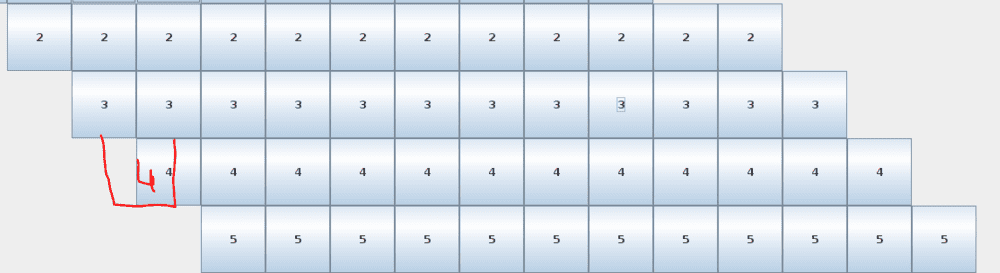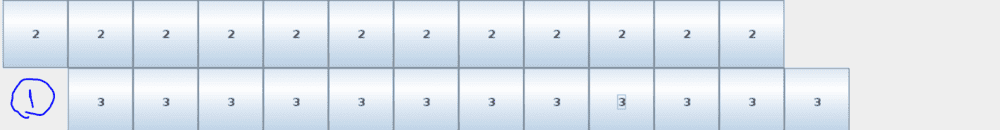# JButton in JPanel with gridlayout

• Comp Sci
anonim
Homework Statement:
Gui game
Relevant Equations:
-
Is it possible to bring it into this shape using the gridlayout?I use empty label to add this empty gridThis is my code:

Java:
import javax.swing.*;
import java.awt.*;
public class test {
public static void main(String[] args) {
JFrame frame = new JFrame();
frame.setTitle("Buttons");
frame.setSize(800, 800);
int size=12;
int flag1=0,count=0,count2=0;
frame.setLayout(new GridLayout(1,2,0,0));
JPanel panel = new JPanel();
GridLayout layout = new GridLayout(size,(size*2),0,0);
panel.setLayout(layout);

for(int i=1; size>=i; i++){
for(int k=0; i-1>k; k++){
count2++;
}

for(int k=0; size>k; k++){
JButton button = new JButton(""+i);
button.setBackground(Color.WHITE);
count2++;
}

for(int k=0; (size*2)-(count2)>k; k++){
flag1=1;
}
if(flag1==1){
count2=0;
flag1=0;
}

}

frame.setVisible(true);
}
}